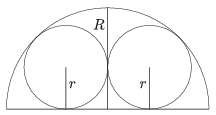Mathematical and Physical Journal
for High Schools
Issued by the MATFUND Foundation
 Already signed up? New to KöMaL?

#Problem B. 4644. (September 2014)

B. 4644. Two circles of radius $\displaystyle r$ are inscribed in a semicircle of radius $\displaystyle R$ such that the circles touch each other externally and the semicircle internally, as shown in the figure. Determine the radius $\displaystyle r$.(3 pont)

Deadline expired on October 10, 2014.

### Statistics:

 308 students sent a solution. 3 points: 221 students. 2 points: 61 students. 1 point: 20 students. 0 point: 5 students. Unfair, not evaluated: 1 solutions.

Problems in Mathematics of KöMaL, September 2014